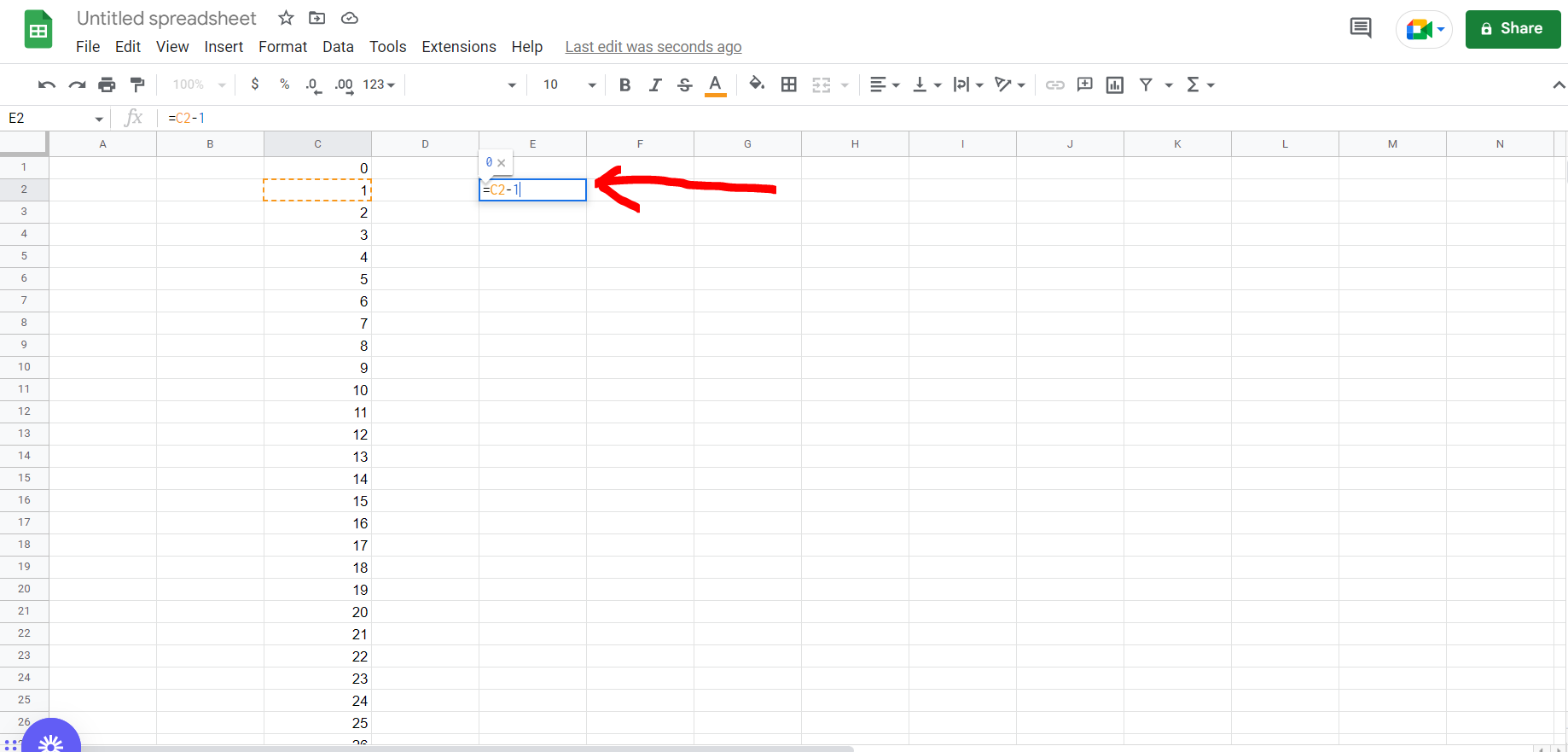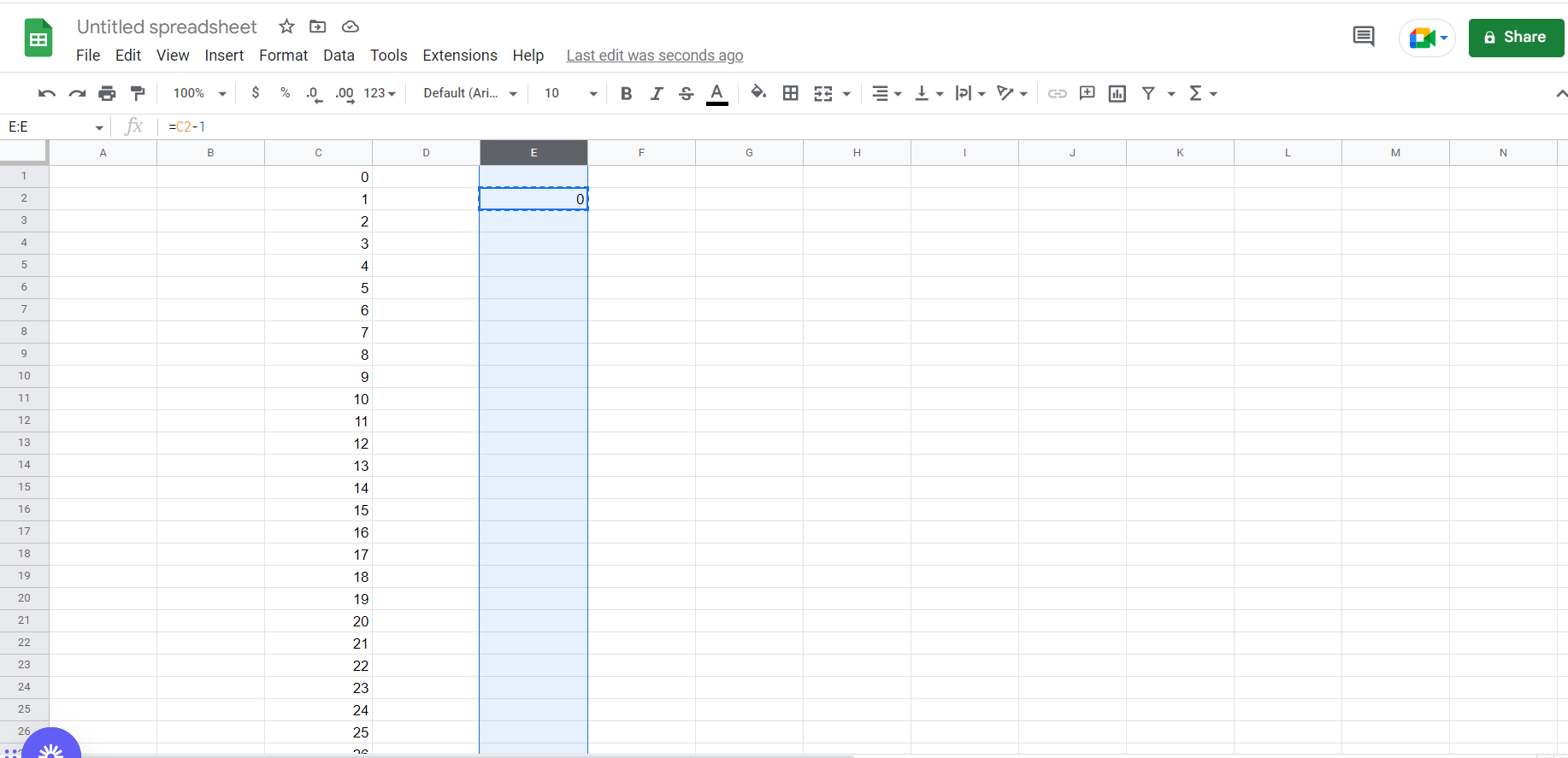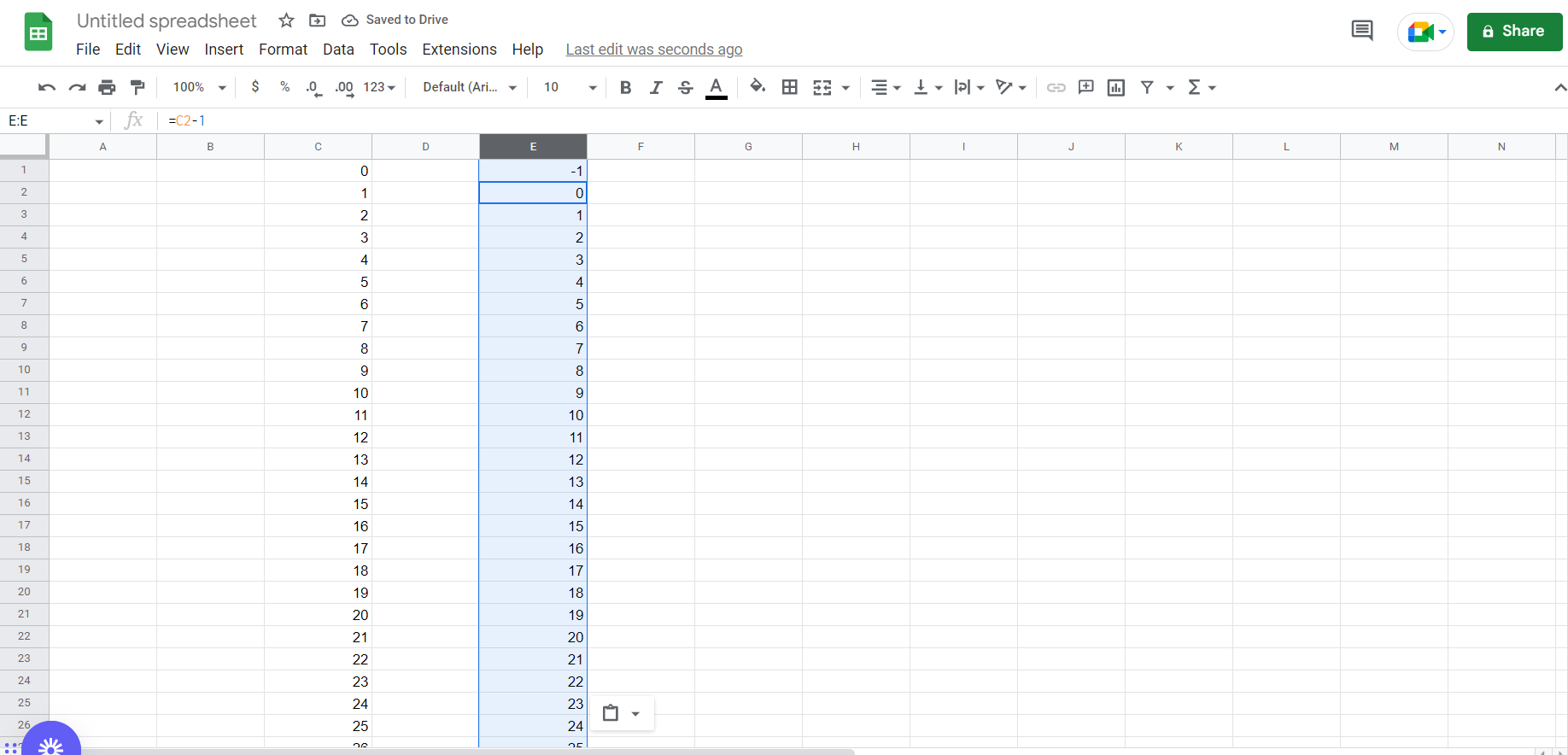# How to apply formula to entire column in Google SheetsGoogle Sheets offer a very interesting way to apply formula to entire column. We can cater to this problem statement by using the CTRL and SPACEBAR keys in google sheets. We can perform the below mentioned way to apply formula to entire column in google sheets:

### Step 1 – Google sheets tab with a list of random values in a column– Open the desired Google Sheets tab containing a column with some values

### Step 2 – Entering the formula for a cell– Now click on any cell and type a random formula for a particular cell in the column, and hit Enter. In our case we have used the “=SUM” formula.

### Step 3 – Copying the formula to the entire column– Now copy the formula we just typed, and then press “CTRL + SPACEBAR”, and then paste the formula.

### Step 4- Formula applied to the entire column– We can see the formula has been applied to the entire column